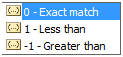# Excel 2D Data Lookup using INDEX with MATCH

INDEX with MATCH enables lookup of a cell in with an unknown reference in a matrix with row and column labels.

The two formulas:

INDEX

Syntax: INDEX(array, row_num, [column_num])

Index is very simple and the syntax is fairly self explanatory.

INDEX takes an array of data as the first parameter, a row number as the second and a column number as the third. The function then return the value in the array referenced by the row and column specified.

An example array in Excel:To return ‘Black’ using the INDEX function use the formula:

=INDEX(B2:D4,3,2)

Note that the second parameter refers to the 3rd row in the array B2:D4 and not the 3rd row of the spreadsheet. This applies for the column reference also.

When used like this, INDEX appears to be fairly limited in its practical applications, as if you already know the row and column number of a cell in the array, you can generally reference the cell directly.

However, when used with MATCH, INDEX becomes one of the most powerful and useful functions in Excel.

MATCH

Syntax: MATCH(lookup_value, lookup_array, [match_type])

MATCH is again quite simple on its own however requires a little more consideration and care than INDEX.

MATCH takes a lookup value as its first parameter and a lookup array as the second. For our purposes the lookup array needs to be a single dimension. This can be row wise or column wise.

MATCH then returns the index of the lookup value if it is found in the lookup array.

However false positives are easy, as the third parameter of MATCH is the [match_type] which takes one of three values:The default value is 1, and this can cause issues as if you forget to add this optional parameter then you could very easily get incorrect lookups occurring that are hard to notice. This is because the lookup will still return a value even if it can not find what you are looking for. MATCH will return some value that is ‘Less than’ the lookup value, which is very rarely what you actually want. It is important when using MATCH with INDEX for matrix lookups to remember to set match_type parameter equal to 0.

An example array in Excel:To return the row index of the array for the value “Colour 2”:

=MATCH(“Colour 2”,A2:A4,0)

This will return: 2

Note that this is the row number of the lookup value “Colour 2” in the lookup array and not in the worksheet.

INDEX with MATCH

MATCH is used to provide logic to the row_num and column_num parameters of INDEX.

If a matrix in Excel has labels for both the rows and columns, then INDEX with MATCH can lookup a specific cell in the matrix with ease.

An example of such a matrix:INDEX with MATCH can be used with this matrix to answer questions such as ‘What is Colour 2 of Group 3?

Repeating from above, the syntax for INDEX is:

INDEX(array, row_num, [column_num])

The input array for this example is B2:D4.

The row_num and column_num parameters need to be found using MATCH as shown above.

row_num: MATCH(“Colour 2”,A2:A4,0) will return 2

col_num: MATCH(“Group 3”,B1:D1,0) will return 3

These can then be put into INDEX to create our formula:

=INDEX(B2:D4,MATCH(“Colour 2”,A2:A4,0),MATCH(“Group 3”,B1:D1,0))

This will return pink.

I now use INDEX with MATCH nearly every time I do data analysis and manipulation in Excel. Clients often have data in 2D Matrices, and it is no longer a hurdle for me to use this data with ease.

Tags:
0 replies